# Time series aggregation

## Comparison of two global averaging approaches

· Statistical Tools,Time series

This post aims to explain and compare two recent and competitive global averaging methods for time series. After introducing the key-ingredients of these two recent methods, we compare them in both clustering and classification tasks.

The complete code generating figures and classification/clustering results can be downloaded here.

What are time series ?

Time series (TS) is a collection of values observed during a given time period at some frequency. We can think about daily financial series such as CAC40, yearly ones such as GNP, daily power consumption series, or weather data such as temperature or pluviometry. As soon as observations are indexed in time order and usually equally spaced, we call such a sequence time series. However, there are also other kind of data that are not really “true times series” but can be transformed into it. For example, DNA, books, shapes and so one.

There are different tasks that can be done with time series and maybe the most current one is the prediction task also well-known as a forecasting problem. However, in this post we are going to focus on averaging a set of time series into one. Such a task is often required for clustering aims (think about kmeans for example) or for visualization aspects: in particular, it enables to summarize and visualize one sequence as the best representant of the considered set of time series. This aggregating process can also be seen as a speed up in executions of statistical processes by decreasing the complexity of computations. For example, in a classification context, using a nearest neighbor algorithm can be very time consuming when the labeled sample size is big. Therefore working with a smaller training dataset based on aggregated times series could speed up the process and be feasible to deal with “real time” data for example.

Averaging time series imply to define a distance and an averaging method and this is the purpose of this post.

Before keeping on reading this post, let us note that all figures and results have been generated via a python code from a 3.5 version which is freely available here. The libraries used to obtain the results come from the tslearn package and the stdw one and need to be installed before running the code. The reader could refer to here and there for an easy installation of packages.

Similarity measure between pairwise of TS

The traditional Euclidean metric is not the most accurate metric on time series for several reasons. Firstly, the order of elements in the sequence has to be taken into account; secondly, the Euclidean distance does not consider neither a phase shift between two curves nor a length difference between series.

There are several similarity measures which have been proposed in the literature to deal with time series, and the reader can have a good overview of the existing ones in the review made by Bagnall et al. In this post we mainly focus on 2 measures which overcome all the limitations described above: the standard elastic distance measure called Dynamic Time Warping (DTW) and the softDTW measure proposed recently by Cuturi and al.

Whereas a one-to-one mapping would be the Euclidean distance between 2 curves, DTW can be viewed as a one-to-many mappings (see example below). Moreover, Soft-DTW is a smoothed version of DTW.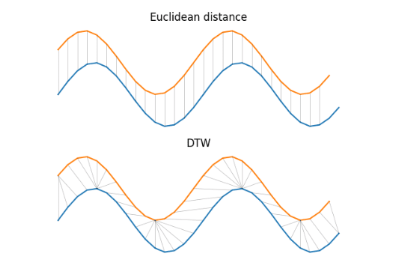More specifically, DTW computes the best global alignement between two sequences of length n and m respectively. In practice, the first nxm pairwise distance matrix are firstly computed and then the coordinates inside both series are aligned. The cost of the optimal alignment is finally computed though a dynamic programming formulation. This can be a very time consuming operation and commonly, constraints are set to the admissible warping paths. For practical considerations, the reader can refer to Mueen et al.).

Instead of considering the optimal alignment which only minimizes the sum of distances between aligned elements, Soft-DTW will consider all possible alignments and retrieve the soft-minimum of the distribution of all costs spanned by all alignments. Note that softDTW depends on a smoothing parameter. When this smoothing parameter is set to 0, the original DTW is recovered. At the opposite, when this smoothing parameter tends to infinity, soft-DTW converges to the sum of all costs.

Whatever approach is chosen, they are both quadratic in time and linear in space complexity according to the length of series. Moreover, both methods can compute a similarity between pairwise of series even if they have different length. However, the ratio of lengths between these pairwise should not exceed twice and be up to ½ to ensure accurate results. Finally, in practice, it is well-recommended to work with normalized data before computing any DTW or soft-DTW distances.

To conclude, let us note that there are several implementations of the standard DTW (in python or in R for example) and soft DTW available in open source.

When data reduction is needed

Aggregation of time series can be seen as a “data reduction” process in the sense that it summarizes a set of time series. In practice, such a process is very used especially in clustering or in classification.

In clustering, most of algorithms iterate 2 main steps: an assignment step and a centering or re-computation step. During the assignment phase, the algorithm computes the distances between each observation and each centroid (also called representant or prototype, which stands for the best representant for the cluster). Then the observation is allocated to its closest cluster (minimum distance with a centroid). During the centering phase, the centroids are updated according to data points assigned to them. In some algorithms such as the well-known kmeans or hierarchical clustering algorithms, the centroids are obtained by aggregating the observations of the same cluster.

In classification, the data reduction is used to reduce the training set into a smaller one. And this is really profitable when kNN-like algorithms are used. Indeed, on one hand, smaller training set may gain accuracy if noisy instances are filtered correctly. On another hand computational complexity decreases when the number of examples in the training set decreases. This is a win-win sketch.

Finally, a third purpose of aggregating time series is a quick and easy way to visualize the main trend of several time series since it summarizes several curves into one (see figure below).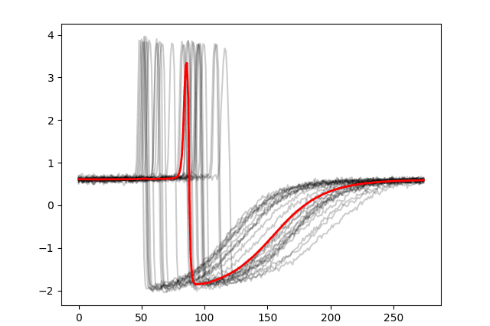Note that we used a sample of the training set of the Trace dataset coming from the UCR time series classification archive. These data have been designed to simulate instrumentation failures in a nuclear power plant. This dataset contains 200 instances, 50 for each class and are made by 275 data points. They are z-normalized. We pick the first class and apply the soft-DTW with a smoothing parameter equals to 1 on the 50 series of this class. A detailed description of this dataset can be found here.

Since we based this post on the DTW distance-like quantity and its soft version, the main question to ask would be: How do we average time series consistently with DTW ? with soft DTW ?

The choice of averaging techniques is really central from an accuracy viewpoint and mainly depends on the end-goal (visualization, classification, clustering, …).

Averaging several time series

Most of averaging algorithms proposed in the literature are based on pairwise averaging methods. Such strategies are really dependent of the order in which the series are taken into account with no guarantee to obtain the same accuracy with a different order. Because of such a limitation, recently two kinds of global approaches have been developed: DTW Barycenter averaging from Petitjean et al. and softDTW from Cuturi and al. The main advantage of these methods is that series are averaged alltogether and therefore there is no impact on the order of consideration of series.

DBA: an approach from Petitjean et al.

This approach aims to compute an “average” sequence, called barycenter, such as the sum of squared DTW between the barycenter and the set of considered sequences is minimum. This is done iteratively and coordinate by coordinate: in other words, each coordinate of the averaged sequence is the barycenter of the associated coordinates of the set of series. Therefore, the sum of squared is minimized locally leading to minimize the total sum of squared DTW similarities.

Soft-DBA: an approach from Cuturi et al.

In this approach, the average sequence is defined as the minimum of a weighted sum of soft-DTW distances between the set of series and the average one. The weights depend on a set of coefficients which can be different for each sequence in the set (but normalized such that their sum equals to 1) and divided by the product of lengths of the considered sequence and the average one.

You can see below an illustration of both approaches on the same sample of data used in the previous paragraph. In blue, the average serie with DBA approach, and in orange (respectively in green) the average sequence computed with soft-DTW barycenter averaging method for a smoothing parameter set to 1 (respectively to 10).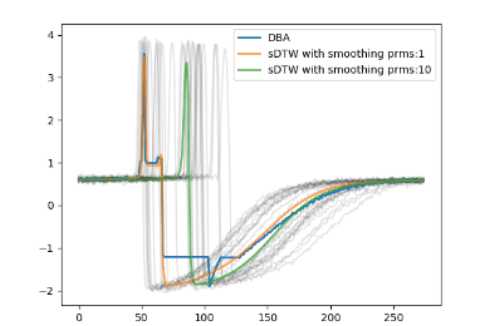Several remarks can be done: firstly, we can notice the effect of the smoothing parameter of the averaged series. Without it, which corresponds to the DBA case, the obtained curve is very granular and it becomes smoother as the smoothing parameter increases. Secondly, in the figure, we can see peaks around the 60th and 100th observations which are not representative of any time series considered in the group. Such a phenomenon highlights the fact that these algorithms can get stuck in local optima, especially when the smoothing parameter is low.

A last practical remark: a bad initialization of averaging method can lead to poor approximations of them. Consequently, in this paper, we use by default the initialization proposed in the tslearn package which is the Euclidean barycenter.

Practical comparisons

We are going to compare the accuracy of both algorithms on two different tasks: clustering and classification. The same data has been used for each approach in goal 1 and goal 2.

The used data (Trace dataset) set is made of 4 classes of time series of length 275 coming from the UCR time series classification archive. These data are z-normalized and splitted into a train and a test sets. In the training set, we dispose of 100 observations, 25 in each class. The test set contains 100 time series.

Goal 1: Clustering task. We apply kmeans-like clusterings by using DBA and soft-DTW barycenter averaging methods on the training set without taking into account the labels. However, we set the number of clusters to 4, equals to the true number of classes.

In order to evaluate the accuracy of clustering, we use the adjusted rand index (ARI) which measures the similarity between two partitions of data by counting pairwise of observations that are assigned in the same or different clusters. When ARI is equal to 1, both partitions match perfectly; when it tends to 0, it means that we have completely different repartition of pairs of data. We apply ARI between the true partition of data (labels of the train set) and the ones obtained by the 3 approaches described below:

1 – km_DBA: kmeans based on DTW distance and DBA as averaging method.

2 – km_sDTW_1: kmeans based on soft-DTW distance and soft-DTW averaging with a smoothing parameter equals to 1.

3 – km_sDTW_10: kmeans based on soft-DTW distance and soft-DTW averaging with a smoothing parameter equals to 10.

It is well-known that the quality of the clustering results is really dependant of the initialization. That is why we used the same random initialisations for all approaches and iterate each experience 4 times.

Moreover, for all approaches, the number of iterations of kmeans has been fixed to 100, and also the ones linked to the computation of barycenters to 100. The number of clusters is set to 4.

The results below present for each approach the ARI averaged on the 4 experiences and its standard deviation.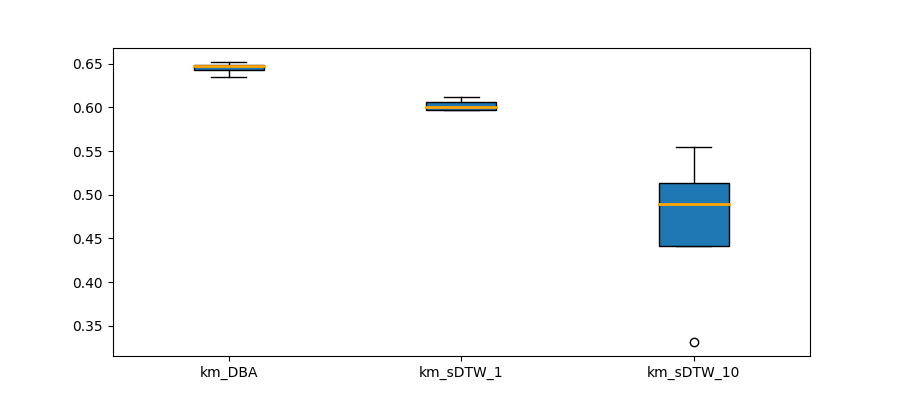As we can observe, on this studied dataset, the first method based on DBA seems to be the most appropriate and the most stable. The average ARI is 0.64 with a small variability 0.006. For the soft-DTW approaches, in the less smoothest case (smoothing parameter set to 1), the average ARI is 0.60 whereas in the smoothest one (with parameter set to 10), the average is about 0.47 with a larger standard deviation (0.08).

We have illustrated in the figure below, an example of 4 centroids fitted for each cluster by each approach.Goal 2: Classification accuracy: each class of the training set is summarized by one time serie as its barycenter and a 1-NN classification is done on the test set. A classification accuracy is computed with the labels in the test set.

Note that we could have done a clustering first on each class in order to summarize all the heterogeneity of the class and then proceed to a 1-NN on these representants. However, in a sake of simplicity, we compute an average representant for each class in a train set of the studied datasets and compute an accuracy error for each tested methods.

We used the following averaging approaches before the 1-NN classifier:

1 – DBA from the DTW distance

2 – soft-DTW averaging based on the soft-DTW distance with smoothing parameter set to 1.

3 – soft-DTW averaging based on the soft-DTW distance with smoothing parameter set to 10.

Again, the initialization of all averaging methods is Euclidean barycenter-based. As a result, we obtain the following classification accuracy: 0.97, 0.74 and 0.72 of good classification rate for the approach 1, 2 and 3 respectively. On this particular dataset, averaging time series with DBA in a classification context seems to be the best method. However, in order to have a better view of performances of both approaches, we are going to test them on several datasets according to a similar process.

Goal 3: Classification accuracy on 12 sets of the UCR datasets. The UCR time series classification archive is a superset of time series data from which we dispose of classification errors computed via the Euclidean and DTW distances and also the state-of-the art algorithm as a reference.

The considered datasets are the following: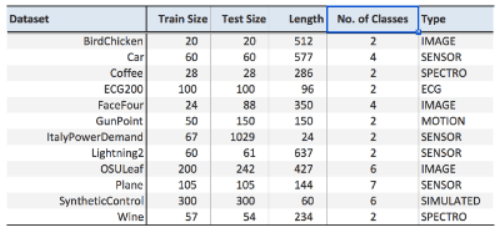As you can see, the 12 datasets used in this post differ from their size of the training and test sets, their length, their number of classes and also their type.

This time, each class of the training set is summarized by 5 time series. They are built by randomly picked a set of series in the same class and averaged according to the proposed methods described in goal 2. A 1-NN classification is done on the test set according to these barycenters : we assign each time series of the test set in the class associated to the closest barycenter. This process is iterated 10 times for each dataset. As previously, we consider 3 approaches: DBA and soft-DTW barycenter averaging approach with smoothing parameters equal to 1 and 10.

The Figure below plots the averaged accuracy obtained by using DBA according to the maximum averaged accuracy obtained with Soft-DTW barycenter on the 12 test sets. When the points are below the diagonal, it implies that DBA provides better classification rates than soft DTW barycenter. When they are above, this is the last method which is the best.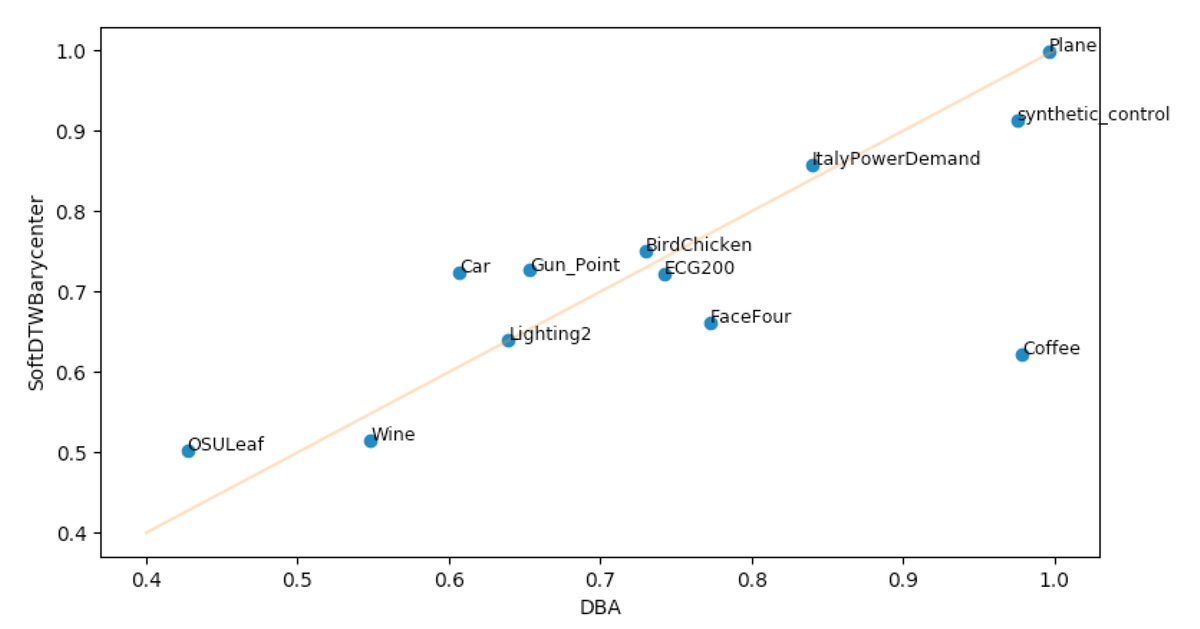Globally, there is no rule: on half of datasets, DBA seems to provide better classification results than Soft-DTW barycenter averaging method. For the remaining datasets, we have the opposite results. There are some datasets however for which DBA outperforms such as on the Coffee data and also the synthetic control one. However, we need to nuance the results of this study. Indeed, firstly, the smoothing parameter for the soft-DTW averaging approach has not been optimized on the training set (we set it to 1 and 10) and this calibrating process could lead to improve associated classification results. Secondly, the DTW distance disposes also of computational tricks and parameters to calibrate that have not been investigated for a sake of "clarity" of this post. Finally, instead of picking randomly series in each class and averaging them, we could have done a clustering on series of the same class (ie per class) in order to better catch the variability intra-class of sequences before averaging them. This could lead to better prototypes (barycenters) for each class and could improve the classification task.

Conclusion

In this post, we have seen two recent and global competitive methods to average time series. However, there are lots of variants and tricks you need to investigate .

Last remark: time series can also be seen as a discretization of functions of time and an alternative way to deal with them is based on functional data analysis. The reader could refer to this previous post written by Matthieu Saumard for a quick and practical start on these techniques and to Ramsay and Silverman as an excellent reference of recent works on functional data.

All Posts
×

Almost done…

We just sent you an email. Please click the link in the email to confirm your subscription!